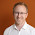## Jun 12, 2012

### HyperLogLog C# implementation

Hi,

Ever wanted to count distinct things with small amounts of ram? Well the guys found an algorithm and called it "LogLog". Furthermore, a newer version called HyperLogLog arrived with some additional goodies. Pros: Speed and minimum use of Ram, Cons: The output is not exact, rather approximate.

I met LogLog and HyperLogLog while searching for the distinct problem here:
http://stackoverflow.com/questions/5990713/loglog-algorithm-for-counting-of-large-cardinalities

The js version of the user @actual was nice and clean, so I tried to convert it into C#. Tested with real life data of size ~1.2 million, the result was 91 to 99 percent correct. But with amazing speed!

Sample usage (Windows forms):
```string[] words = {"sample","keywords"}; // 1.200.000 other strings.

foreach (string word in words)
log_log.Add(word);
this.Text = string.Format("{0} calculated distinct items. ", log_log.Count());
```

```public class HyperLogLog
{
private double mapSize, alpha_m, k;
private int kComplement;
private Dictionary<int, int> Lookup = new Dictionary<int, int>();
private const double pow_2_32 = 4294967297;

public HyperLogLog(double stdError)
{
mapSize = (double)1.04 / stdError;
k = (long)Math.Ceiling(log2(mapSize * mapSize));

kComplement = 32 - (int)k;
mapSize = (long)Math.Pow(2, k);

alpha_m = mapSize == 16 ? (double)0.673
: mapSize == 32 ? (double)0.697
: mapSize == 64 ? (double)0.709
: (double)0.7213 / (double)(1 + 1.079 / mapSize);
for (int i = 0; i < mapSize; i++)
Lookup[i] = 0;
}

private static double log2(double x)
{
return Math.Log(x) / 0.69314718055994530941723212145818;//Ln2
}
private static int getRank(uint hash, int max)
{
int r = 1;
uint one = 1;
while ((hash & one) == 0 && r <= max)
{
++r;
hash >>= 1;
}
return r;
}
public static uint getHashCode(string text)
{
uint hash = 0;

for (int i = 0, l = text.Length; i < l; i++)
{
hash += (uint)text[i];
hash += hash << 10;
hash ^= hash >> 6;
}
hash += hash << 3;
hash ^= hash >> 6;
hash += hash << 16;

return hash;
}

public int Count()
{
double c = 0, E;

for (var i = 0; i < mapSize; i++)
c += 1d / Math.Pow(2, (double)Lookup[i]);

E = alpha_m * mapSize * mapSize / c;

// Make corrections & smoothen things.
if (E <= (5 / 2) * mapSize)
{
double V = 0;
for (var i = 0; i < mapSize; i++)
if (Lookup[i] == 0) V++;
if (V > 0)
E = mapSize * Math.Log(mapSize / V);
}
else
if (E > (1 / 30) * pow_2_32)
E = -pow_2_32 * Math.Log(1 - E / pow_2_32);
// Made corrections & smoothen things, or not.

return (int)E;
}

public void Add(object val)
{
uint hashCode = getHashCode(val.ToString());
int j = (int)(hashCode >> kComplement);

Lookup[j] = Math.Max(Lookup[j], getRank(hashCode, kComplement));
}
}
```

#### 2 comments:

1.Thanks for this post! You might want to check out this more recent HyperLogLog implementation:
https://github.com/Microsoft/CardinalityEstimation

It has some improvements, making it more accurate and memory-efficient than the above, especially for small cardinalities.And it only took 2.5 extra years ;)

2.Well well well, I didn't expect less from Microsoft anyways.

Nice work!

slm asl?